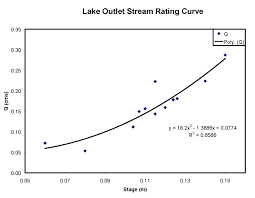## How to Calculate and Solve for Discharge | Slope Area Method | Water BudgetThe image above represents discharge | slope area method.

To compute for discharge | slope area method, four essential parameters are needed and these parameters are Manning’s Co-efficient (N), Area (A), Hydraulic Radius (R) and Slope (S).

The formula for calculating discharge | slope area method:

Q = (A / N) R2/3 S1/2

Where;

Q = Discharge
N = Manning’s Co-efficient
A = Area
S = Slope

Let’s solve an example;
Find the discharge when the manning’s co-efficient is 4, the area is 30, the hydraulic radius is 15 and the slope is 22.

This implies that;

N = Manning’s Co-efficient = 4
A = Area = 30
R = Hydraulic Radius = 15
S = Slope = 22

Q = (A / N) R2/3 S1/2
Q = (30 / 4) (15)2/3 (22)1/2
Q = (7.5) (6.08) (4.69)
Q = 213.96

Therefore, the discharge is 213.96.